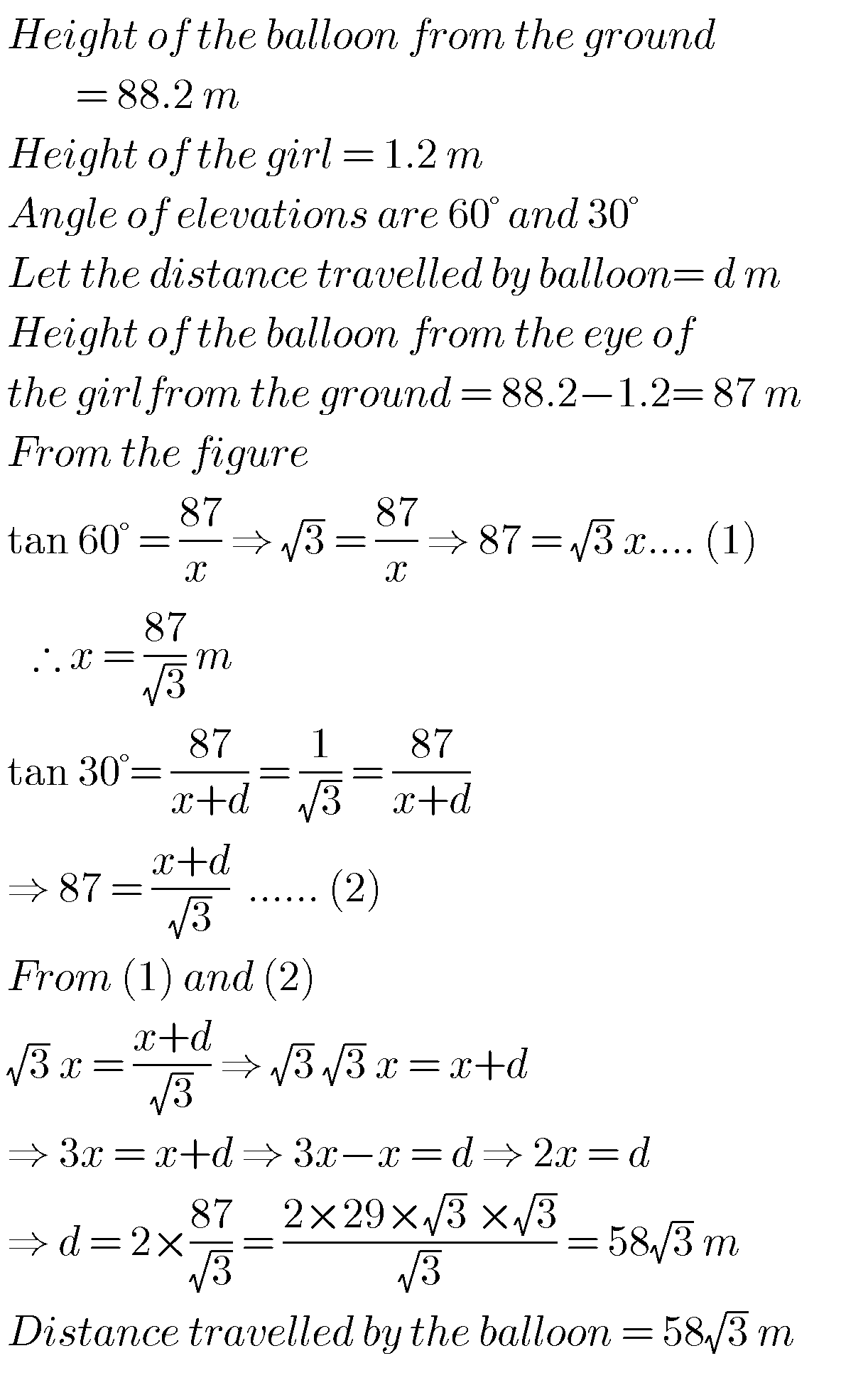## Maths solutions for Applications of Trigonometry class 10 ssc

Maths solutions for Applications of Trigonometry class 10 ssc Tenth class mathematics chapter 12 exercises 12.1 and 12.2 solutions are given.  These solutions are very easy to understand. First study the chapter 12 well for many times. Observe the example probems and solutions well. Try them in your own method. Next solve the exercise problems. …